Next: Ehrenfest's Theorem Up: Fundamentals of Quantum Mechanics Previous: Normalization of the Wavefunction

# Expectation Values and Variances

We have seen that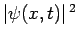is the probability density of a measurement of a particle's displacement yielding the valueat time. Suppose that we made a large number of independent measurements of the displacement on an equally large number of identical quantum systems. In general, measurements made on different systems will yield different results. However, from the definition of probability, the mean of all these results is simply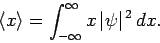(158)

Here,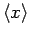is called the expectation value of. Similarly the expectation value of any function ofis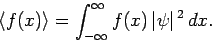(159)

In general, the results of the various different measurements ofwill be scattered around the expectation value. The degree of scatter is parameterized by the quantity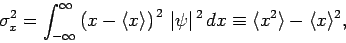(160)

which is known as the variance of. The square-root of this quantity,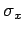, is called the standard deviation of. We generally expect the results of measurements ofto lie within a few standard deviations of the expectation value.

For instance, consider the normalized Gaussian wave packet [see Eq. (146)]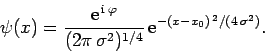(161)

The expectation value ofassociated with this wavefunction is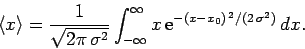(162)

Let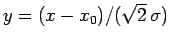. It follows that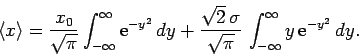(163)

However, the second integral on the right-hand side is zero, by symmetry. Hence, making use of Eq. (144), we obtain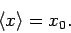(164)

Evidently, the expectation value offor a Gaussian wave packet is equal to the most likely value of(i.e., the value ofwhich maximizes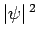).

The variance ofassociated with the Gaussian wave packet (161) is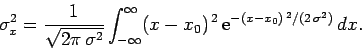(165)

Let. It follows that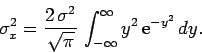(166)

However,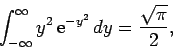(167)

giving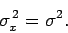(168)

This result is consistent with our earlier interpretation of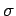as a measure of the spatial extent of the wave packet (see Sect. 3.12). It follows that we can rewrite the Gaussian wave packet (161) in the convenient form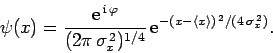(169)Next: Ehrenfest's Theorem Up: Fundamentals of Quantum Mechanics Previous: Normalization of the Wavefunction
Richard Fitzpatrick 2010-07-20